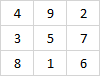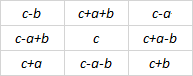# Magic square rows and columns as numbers

Take any 3 by 3 magic square. For example, here’s the ancient Lo Shu square:If you read the rows as numbers and sum their squares, you get the same thing whether you read left to right or right to left. In this case

4922 + 3572 + 8162 = 2942 + 7532 + 6182.

Similarly, if you read the columns as numbers and sum their squares, you get the same thing whether you read top to bottom or bottom to top:

4382 + 9512 + 2762 = 8342 + 1592 + 6722.

This doesn’t depend on base 10. It’s true of any base. And the entries of the magic square do not have to be single digits as long as you take the first to be the coefficient of b2, the second the coefficient of b, and the last the coefficient of 1, where b is your base.

In addition to rows and columns, you can get analogous results for diagonals.

4562 + 9782 + 2312 = 6542 + 8792 + 1322

4562 + 3122 + 8972 = 6542 + 2132 + 7982

2582 + 9362 + 4712 = 8522 + 6392 + 1742

2582 + 7142 + 6932 = 8522 + 4172 + 3962

How would you prove this? Arthur Benjamin and Kan Yasuda give an elegant proof here using permutation matrices. Or you could use brute-force starting with Édouard Lucas’ theorem that every 3 by 3 magic square has the following form.(For each ab, and c there are eight variations on magic square given by Lucas, reflections and rotations of his square.)

Benjamin and Yasuda attribute this discovery to R. Holmes in 1970. “The magic magic square”, The Mathematical Gazette, 54(390):376.## 2 thoughts on “Magic square rows and columns as numbers”

1. David Tate

I shared an office with Art Benjamin in the summer of 1984, when we were both students working at the National Bureau of Standards (later NIST). It has been fun to follow his career.